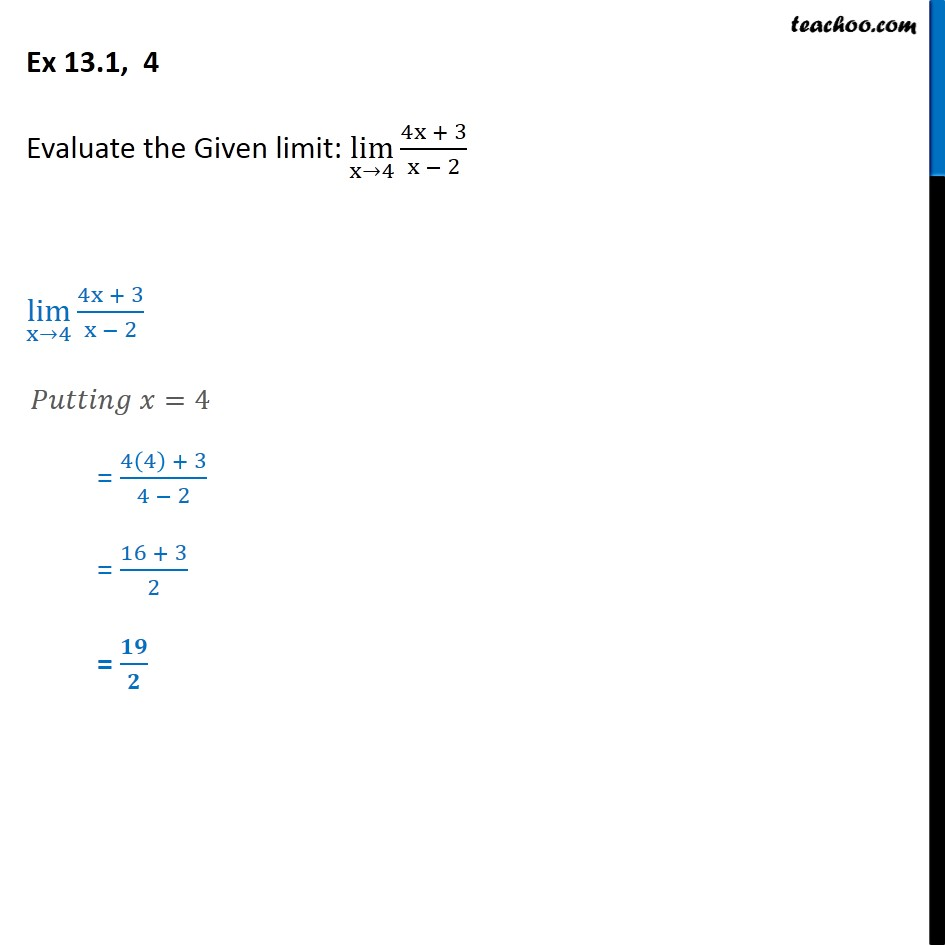1. Chapter 13 Class 11 Limits and Derivatives
2. Serial order wise
3. Ex 13.1

Transcript

Ex 13.1, 4 - Chapter 13 Class 11 Limits and Derivatives - NCERT Evaluate the Given limit: lim (x →4)⁡ [(4x + 3)/(x − 2)] lim (x→4)⁡〖(4x + 3)/(x − 2)〗 Putting x = 4 = (4(4) + 3)/(4 − 2) = (16 + 3)/2 = 19/2

Ex 13.1

About the AuthorDavneet Singh
Davneet Singh is a graduate from Indian Institute of Technology, Kanpur. He has been teaching from the past 9 years. He provides courses for Maths and Science at Teachoo.